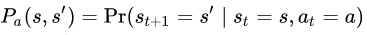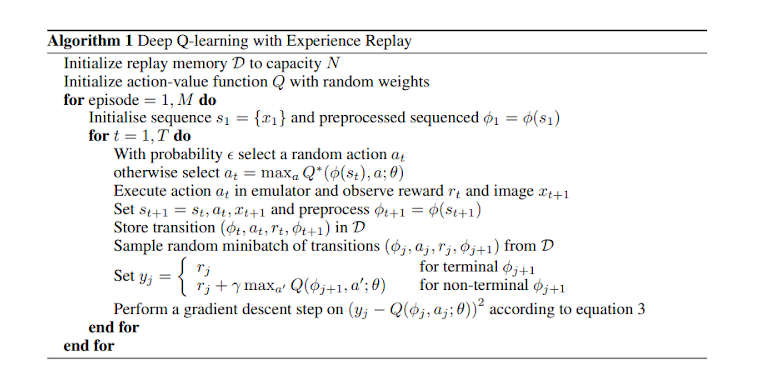# 1. GOAL

To implement different RL algorithms from scratch and test them on a Highway Environment  developed using OpenAI gym

# 2. MOTIVATION

The ‘highway-env’ is an environment in the OpenAI gym, which has different scenarios of autonomous driving. We are going to choose the ‘parking’ environment wherein the goal is to make sure the ego-vehicle is parked at the desired space with the appropriate heading. The motivation for this is the theme ‘Habitat AI challenge: Point Navigation’, wherein the agent has to efficiently navigate from one point to another under various constraints. Similar to that, in this problem, the vehicle needs to navigate from an initial location to the parking point while achieving an appropriate orientation.

# 3.1. Markov Decision Process (MDP)

A Markov decision process is a 4-tuple

• S is a set of states called the state space,
• A is the action space and can be considered as a set of all possible actions
• Rₐ(s, a) is the immediate reward (or expected immediate reward) received after transitioning from state s to state s’, due to action athe probability that action a in state s at time t will lead to state s’ at time t+1

# Model-Based Reinforcement Learning

## Principle

With a known reward function R and subject to unknown deterministic dynamics

1. Model learning: A model of the dynamics f_θ≃f is learned through a regression on interaction data.
2. Planning: We then leverage the dynamics model f_θ to compute the optimal trajectory

## Motivation

• Sparse rewards
• The complexity of the policy/value vs dynamics

Is it easier to decide which action is best, or to predict what is going to happen?

• Some problems can have complex dynamics but a simple optimal policy or value function. For instance, consider the problem of learning to swim. Predicting the movement requires understanding fluid dynamics and vortices while the optimal policy simply consists of moving the limbs in sync.
• Conversely, other problems can have simple dynamics but complex policies/value functions. Think of the game of Go, its rules are simplistic (placing a stone merely changes the board state at this location) but the corresponding optimal policy is very complicated.
• Inductive bias
• Sample efficiency
• Interpretability

## Approach

We consider the parking-v0 task of the highway-env environment. It is a goal-conditioned continuous control task where an agent drives a car by controlling the gas pedal and steering angle and must park in a given location with the appropriate heading.

• The policy/value is highly dependent on the goal which adds a significant level of complexity to a model-free learning process, whereas the dynamics are completely independent of the goal and hence can be simpler to learn.
• In the context of an industrial application, we can reasonably expect for safety concerns that the planned trajectory is required to be known in advance, before execution.
• Draw samples from a probability distribution. We use Gaussian distributions over sequences of actions.
• Minimize the cross-entropy between this distribution and a target distribution to produce a better sample in the next iteration. We define this target distribution by selecting the top-k performing sampled sequences.

## Results

Below is the graph to depict the training and validation loss vs epochs for step 3, in which the

The algorithm and math is all fine, but can the MDP park the car now?

## 3.2 Deep Q Learning Network (DQN)

In traditional Q learning, a table is constructed to learn state-action Q values. Given a current state, the goal is to learn a policy that aids the agent in taking an action that results in maximizing the total reward. It is an off-policy algorithm i.e. the function learns from random actions that are outside the current policy. This can however be expensive in terms of memory and computation.DQN Algorithm. Reference: Mnih et al 
1. 3 steering actions, 3 accelerations, hidden state = 128, 4 layers, gamma 0.1 — the network overfits
2. 5 steering, 2 accelerations, hidden state = 128, 4 layers, gamma 0.1 — the network overfits
3. 5 steering, 2 accelerations, 3 layers, hidden size 128 — the network overfits

# 3.3. Soft Actor-Critic (SAC)

Soft Actor-Critic (SAC) is a model-free — off policy deep Reinforcement Learning algorithm. SAC has properties that make it desirable over a lot of existing deep Reinforcement algorithms. These properties are,

• Sample Efficiency: In general, learning skills in the real world can take a huge amount of time. Thus, adding a new task to the agent will require several trials. But compared to other algorithms, SAC has a good sample efficiency which makes learning new skills comparatively faster.
• Lack of Sensitive Hyperparameters: In real-world scenarios, tuning the hyperparameters regularly is not ideal and we need a model that is not very sensitive to hyperparameters.
• Off — Policy Learning: In general, an algorithm is said to be off-policy if the data collected for a different task can be used for another purpose. SAC being an off-policy algorithm makes it easier to adapt it for other tasks just by tuning the reward function and parameters accordingly.
1. n_sampled_goal=4
2. goal_selection_strategy=’future’
3. verbose = 1
4. buffer_size=int(1e6)
5. Learning rate=1e-3
6. gamma=0.9
7. Batch size=256
8. Training steps = 5e4

# 3.4. DEEP DETERMINISTIC POLICY GRADIENT (DDPG)

Deep Deterministic Policy Gradient (DDPG) is a Reinforcement Learning technique that is used in environments with continuous action spaces and is an improvement over the vanilla Actor-Critic (A2C) algorithm. It is an “off”-policy method which combines both Q-learning and Policy gradients.

1. Q network: The Q-value network or critic takes in state and action as input and outputs the Q-value.
2. Deterministic policy network: The policy network or actor takes the state as input and outputs the exact action (continuous), instead of a probability distribution over actions. Thus, DDPG works in deterministic settings.
3. Target Q network: It is a time-delayed copy of the original Q network.
4. Target policy network: It is a time-delayed copy of the original policy network.
1. Actor learning rate=1e-4
2. Critic learning rate=1e-3
3. gamma=0.99
4. tau=0.005
5. Batch size = 128
6. Hidden size (in all 4 networks) = 256
7. Maximum memory size = 50000
8. Steps per episode = 500
1. n_sampled_goal=4
2. goal_selection_strategy=’future’
3. online_sampling=True
4. buffer_size=int(1e6)
5. Learning rate=1e-3
6. gamma=0.95
7. Batch size=256
8. max_episode_length=100
9. noise_std = 0.2
10. Training steps = 2e5

# 4. CHALLENGES:

Getting algorithms to work in the continuous action space was hard. This is a newly defined environment and hasn’t been explored much, which led to difficulty in hyperparameter tuning.

• Model bias
• The computational cost of planning

# 5. REFERENCES:

1. https://github.com/eleurent/highway-env
2. https://en.wikipedia.org/wiki/Ornstein%E2%80%93Uhlenbeck_process
3. Timothy P. Lillicrap, Jonathan J. Hunt, Alexander Pritzel, Nicolas Heess, Tom Erez, Yuval Tassa, David Silver, and Daan Wierstra, Continuous control with deep reinforcement learning, CoRR abs/1509.02971 (2015)
4. Edouard Leurent’s answer to Quora's postWhy do we use the Ornstein Uhlenbeck Process in the exploration of DDPG?”
5. https://towardsdatascience.com/reinforcement-learning-with-hindsight-experience-replay-1fee5704f2f8
6. Hindsight Experience Replay, Andrychowicz et al., 2017